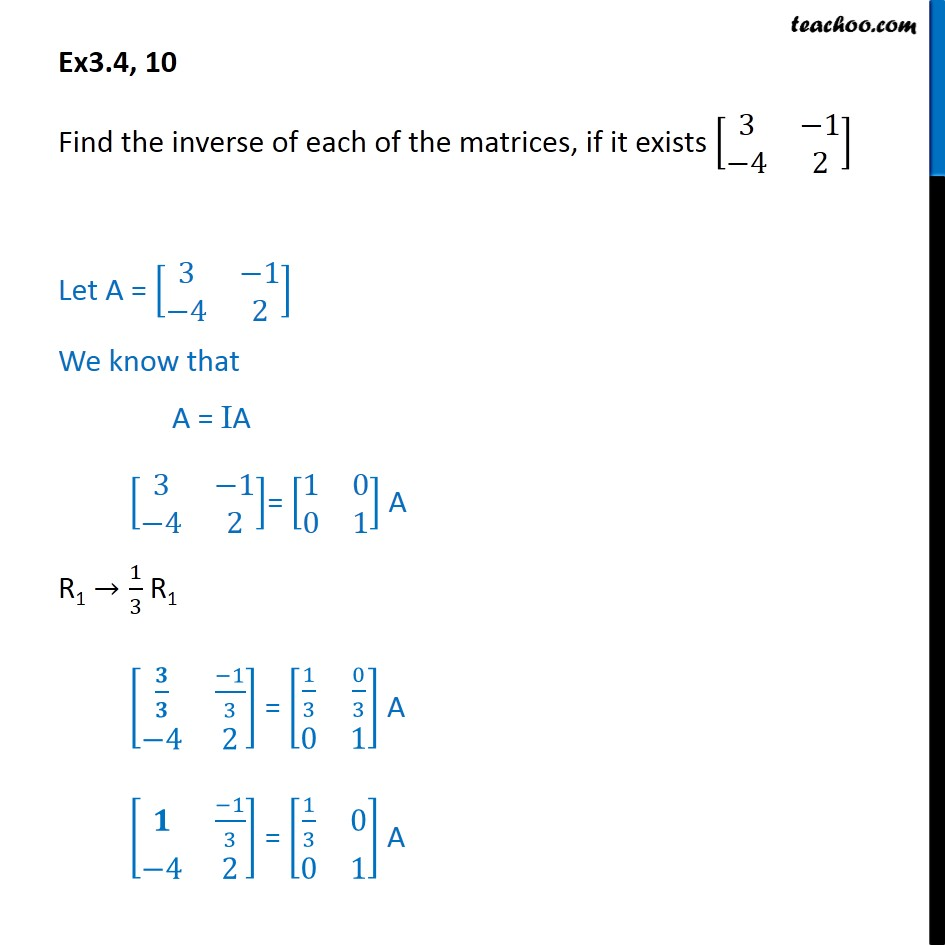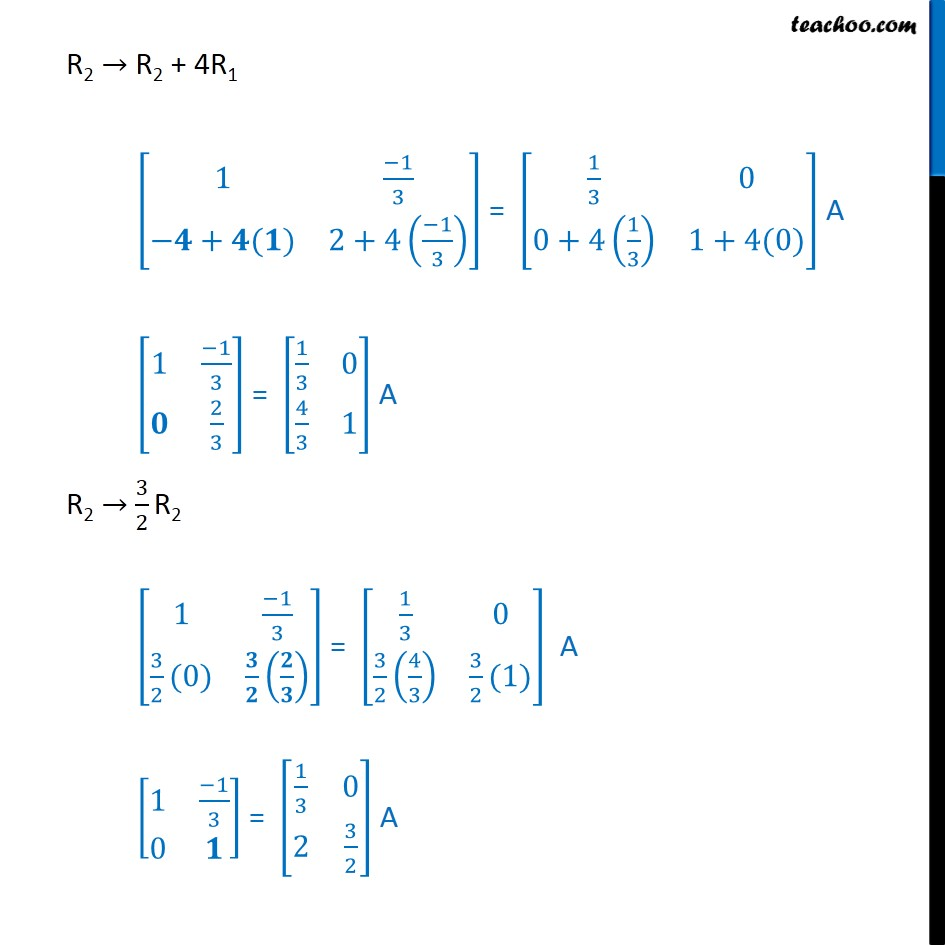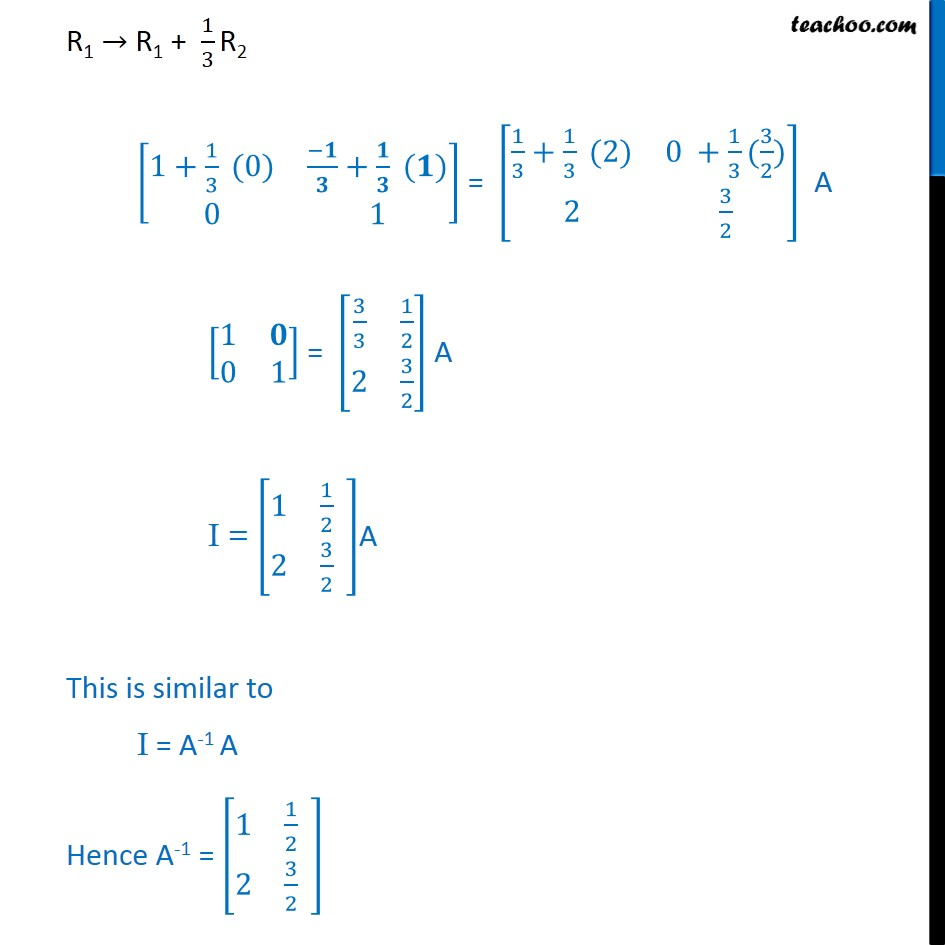1. Chapter 3 Class 12 Matrices
2. Concept wise
3. Inverse of matrix using elementary transformation

Transcript

Ex3.4, 10 Find the inverse of each of the matrices, if it exists [■8(3&−1@−4&2)] Let A = [■8(3&−1@−4&2)] We know that A = IA [■8(3&−1@−4&2)]= [■8(1&0@0&1)] A R1 →1/3 R1 [■8(𝟑/𝟑&(−1)/3@−4&2)] = [■8(1/3&0/3@0&1)] A [■8(𝟏&(−1)/3@−4&2)] = [■8(1/3&0@0&1)] A R2 → R2 + 4R1 [■8(1&(−1)/3@−𝟒+𝟒(𝟏)&2+4((−1)/3) )] = [■8(1/3&0@0+4(1/3)&1+4(0))] A [■8(1&(−1)/3@𝟎&2/3)] = [■8(1/3&0@4/3&1)] A R2 → 3/2 R2 [■8(1&(−1)/3@3/2(0)&𝟑/𝟐 (𝟐/𝟑) )] = [■8(1/3&0@3/2 (4/3)&3/2(1))] A [■8(1&(−1)/3@0&𝟏)] = [■8(1/3&0@2&3/2)] A R1 → R1 + 1/3 R2 [■8(1+1/3 (0)&(−𝟏)/𝟑+𝟏/𝟑 (𝟏)@0&1)] = [■8(1/3+1/3 (2)&0 +1/3(3/2)@2&3/2)] A [■8(1&𝟎@0&1)] = [■8(3/3&1/2@2&3/2)] A I = [■8(1&1/2 " " @2&3/2 " " )]A This is similar to I = A-1 A Hence A-1 = [■8(1&1/2 " " @2&3/2 " " )]

Inverse of matrix using elementary transformation